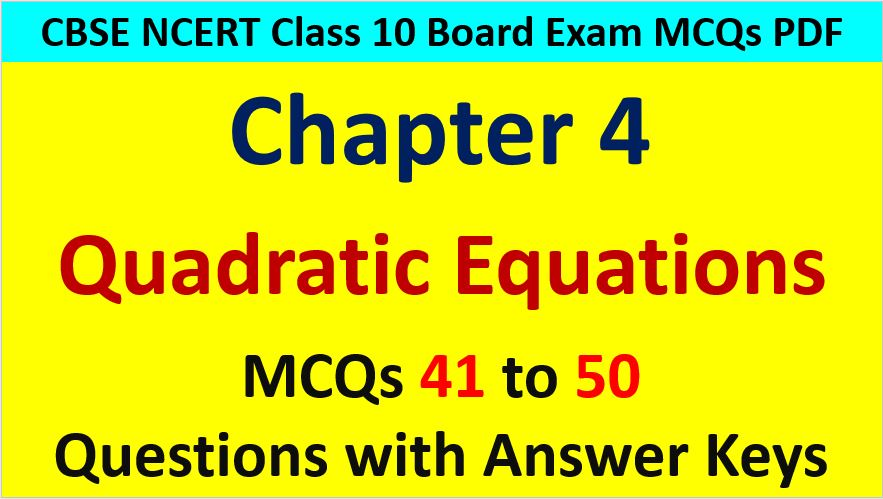Friday, October 22, 2021
Home > CBSE Class 10 > Quadratic Equations CBSE Class 10 Maths MCQ Questions with Answers Keys

# Quadratic Equations CBSE Class 10 Maths MCQ Questions with Answers KeysHi students, Welcome to AMBiPi (Amans Maths Blogs). In this article, you will get Quadratic Equations CBSE Class 10 Maths MCQ Questions with Answer Keys. You can download this PDF and save it in your mobile device or laptop etc.

Quadratic Equations CBSE Class 10 Maths MCQ Question No 41:

If 1 + √2 is a root of a quadratic equation with rational coefficients, then its other root is

Option A : √2

Option B : √2 – 1

Option C : 1/√2

Option D : 1 – √2

Option D : 1 – √2

Quadratic Equations CBSE Class 10 Maths MCQ Question No 42:

If the roots of the equation ax2 + bx + c = 0 be equal, then the value of c is

Option A : b/2a

Option B : –b/2a

Option C : –b2/4a

Option D : b2/4a

Option D : b2/4a

Quadratic Equations CBSE Class 10 Maths MCQ Question No 43:

If one root of the quadratic equation 2x2 + kx – 6 = 0 is 2, find the value of k

Option A : k = -1

Option B : k = 1

Option C : k = 2

Option D : k = 3

Option A : k = -1

Quadratic Equations CBSE Class 10 Maths MCQ Question No 44:

The roots of the equation x2 – 3x + 4 = 0

Option A : Real and equal

Option B : Real and not equal

Option C : Not real

Option D : None of these

Option C : Not real

Quadratic Equations CBSE Class 10 Maths MCQ Question No 45:

The factors of equation 25m2 + 30m + 9 are

Option A : (5m – 3)(5m + 2)

Option B : (5m + 3)(5m – 3)

Option C : (5m – 3)(5m + 3)

Option D : (5m + 3)(5m + 3)

Option D : (5m + 3)(5m + 3)

Quadratic Equations CBSE Class 10 Maths MCQ Question No 46:

Divide 51 into two parts whose product is 378 will be

Option A : 34, 17

Option B : 24, 27

Option C : 42, 9

Option D : 32, 19

Option C : 42, 9

Quadratic Equations CBSE Class 10 Maths MCQ Question No 47:

If 3x2 + 7x – 3 = 0, then the product of the roots is

Option A : -1

Option B : 3/7

Option C : 1

Option D : 7/3

Option A : -1

Quadratic Equations CBSE Class 10 Maths MCQ Question No 48:

If one root of the equation 3x2 – 10x + 3 = 0 is 1/3, then other root is

Option A : –1/3

Option B : -3

Option C : 3

Option D : 1/3

Option C : 3

Quadratic Equations CBSE Class 10 Maths MCQ Question No 49:

If one root of 5x2 + 13x + k = 0 be the reciprocal of the other root, then the value of k is

Option A : 0

Option B : 5

Option C : 2

Option D : 1

Option B : 5

Quadratic Equations CBSE Class 10 Maths MCQ Question No 50:

The two numbers are such that their product is equal to their sum and the numbers are equal. They are

Option A : 11, 11

Option B : 4, 4

Option C : 2, 2

Option D : 3, 3